In two wattmeter method, we have two types of connections:

In star connection of load, the reading of wattmeter one is product phase current and voltage difference (V2-V3) and the reading of wattmeter two is the product of phase current and the voltage difference (V2-V3). Thus, the total power of the circuit is sum of the reading of both the wattmeters.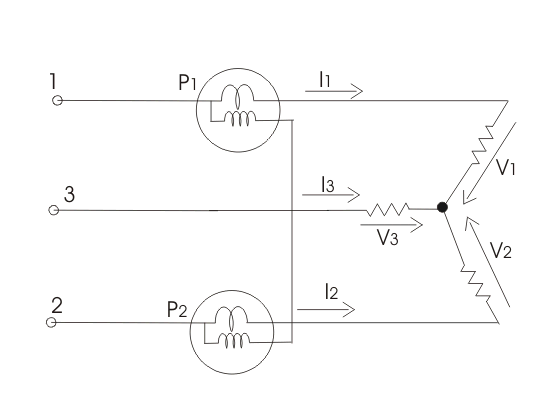Mathematically we can write P=P1+P2=I1(V1+V2) + I2(V2-V3).
But  I1+I2+I3=0, hence putting the value of I1+I2=-I3.
We get total power as P=V1I1+V2I2+V3I3.
In delta connection of loads,  the loads are connected as: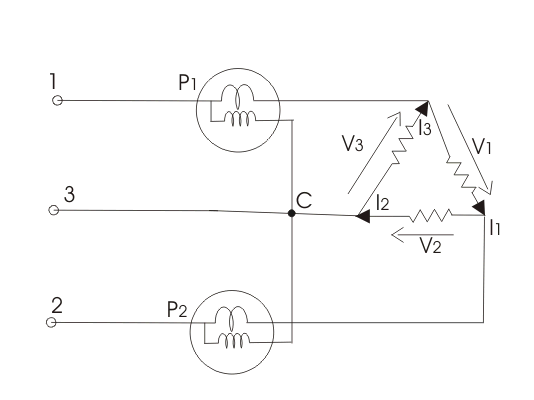The reading of wattmeter one can be written as P1 = -V3(I1 – I3) and reading of wattmeter two is
P2= -V2(I2 – I1).
But V1+V2+V3=0, hence expression for total power is P=V1I1+V2I2+V3I3.

## Practical on Two Wattmeter method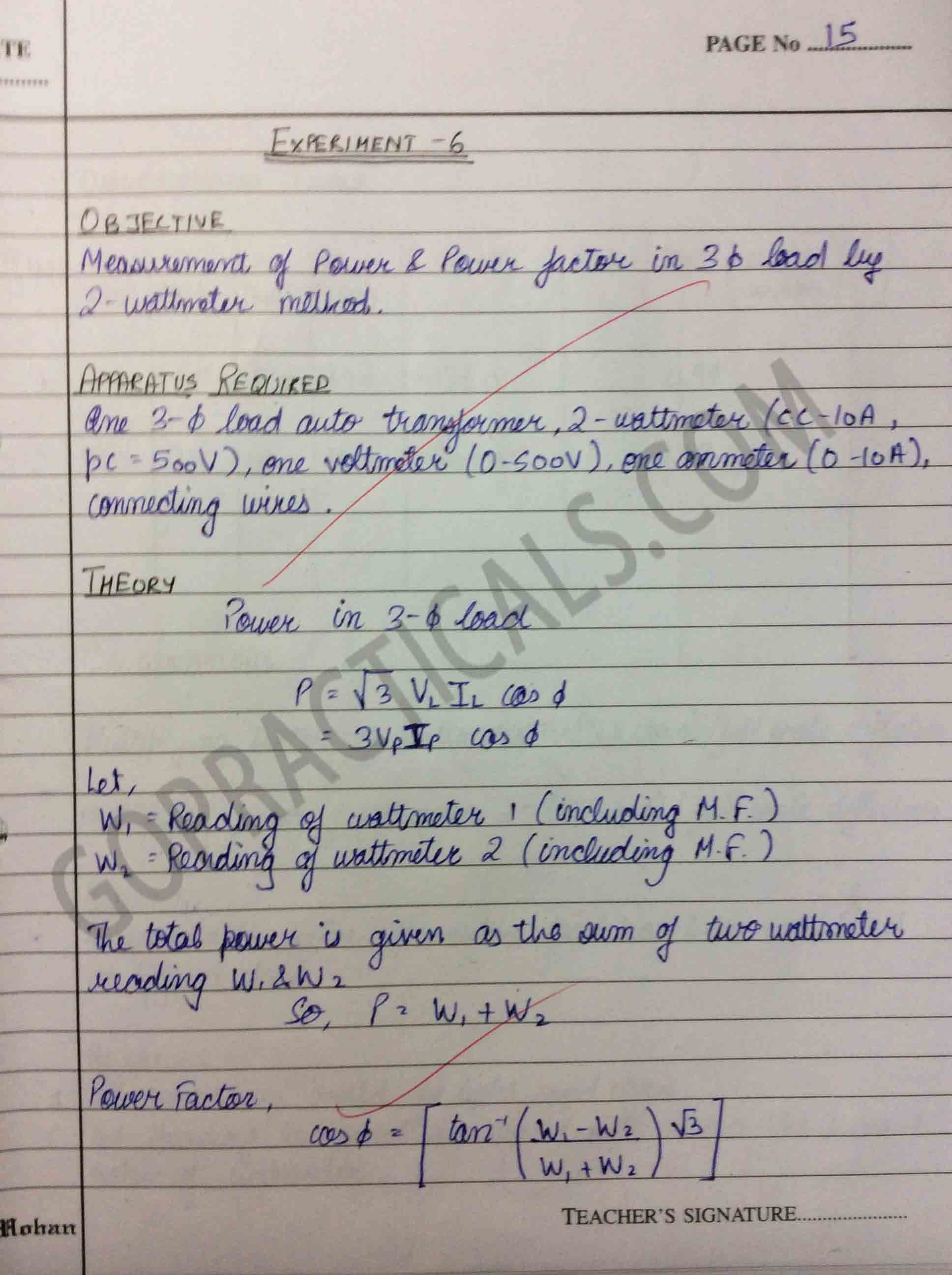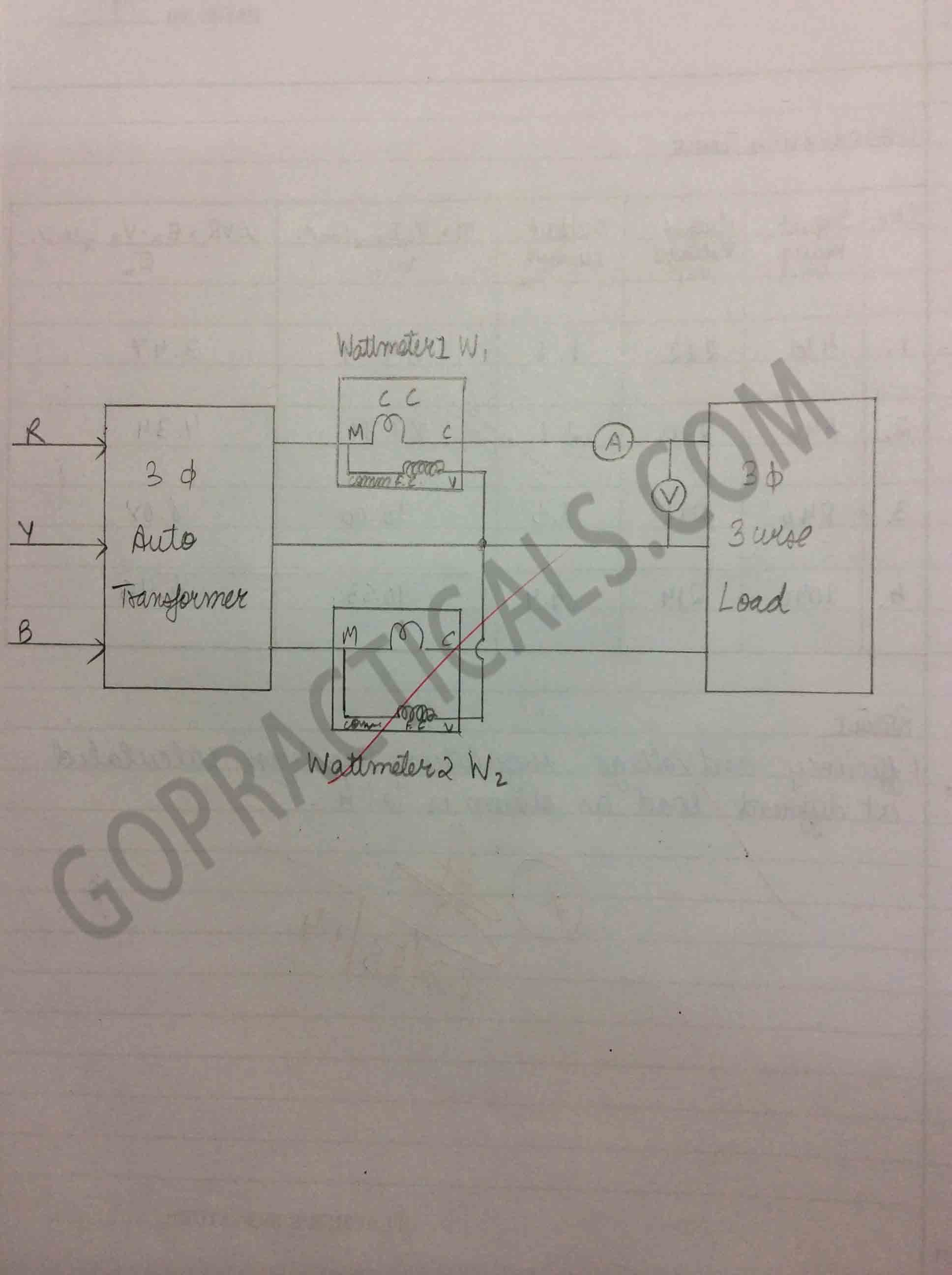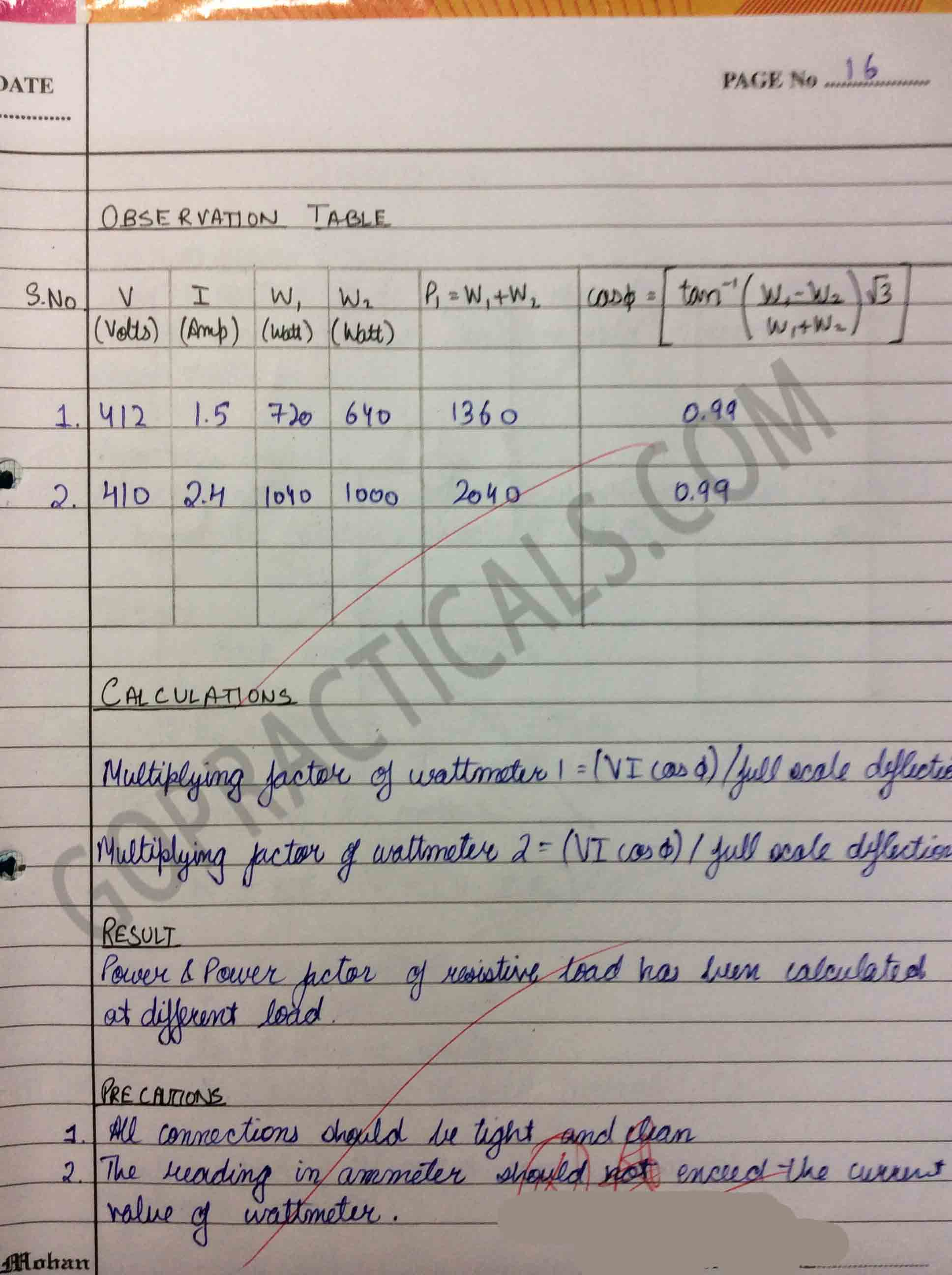## Watch this Video to know about 2 Wattmeter method

Also Check Out – Working of Single Phase Energy Meter

Thanks for viewing our post, hope you liked it and if you think we are helping you then spread the word.

Measurement of Power Factor in 3 Phase Load by 2 Wattmeter Method – Electrical Practical